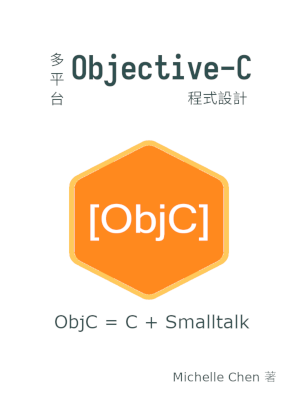#撰寫 Objective-C 的類別 (Class) 和物件 (Object)

## 宣告類別的公開界面

Objective-C 的公開界面的虛擬碼如下：

``````@interface Klass : Base {
/* Instance variable declarations. */
}

/* Public message declarations. */
@end
``````

`@interface``@end` 是固定的語法，公開界面寫在兩者所包起來的區塊中。

Klass 代表此類別的名稱，而 Base 代表所繼承的類別名稱。典型的 Objective-C 類別至少會繼承 `NSObject` 這個共通的基礎類別，完全不繼承任何類別的類別甚少。

Objective-C 的訊息可細分為實體訊息 (instance message) 和類別訊息 (class message)。兩者的差別在傳入的物件是實體 (instace) 還是類別 (class)。另外，類別本身視為特殊物件，所以兩者語法雷同，這是承襲自 Smalltalk 的特性。

``````-(type) instanceMessageWith: (type_a)a andObj: (type_b b;
``````

``````+(type) classMessageWith: (type_a)a;
``````

## 宣告座標點類別 `Point` 的公開界面

``````/* point.h */
#pragma once

#import <Foundation/Foundation.h>

@interface Point : NSObject {
double x;
double y;
}

+(Point *) new;
+(Point *) newWithX: (double)px andY: (double)py;

+(double) distanceBetween: (Point *)p andPoint: (Point *)q;

-(Point *) init;
-(Point *) initWithX: (double) px andY: (double)py;

-(double) x;
-(double) y;
@end
``````

``````@interface Point : NSObject {
double x;
double y;
}
``````

Objective-C 沒有真正為建構子 (constructor) 而設的語法，使用一般的訊息充當建構子即可。本範例程式宣告了四個建構訊息：

``````+(Point *) new;
+(Point *) newWithX: (double)px andY: (double)py;

-(Point *) init;
-(Point *) initWithX: (double) px Y: (double) py;
``````

``````Point *p = [[Point alloc] init];
``````

``````Point *p = [Point new];
``````

``````+(double) distanceBetween: (Point *)p andPoint: (Point *)q;
``````

``````/* p and q are instances of `Point`. */
double dist = [Point distanceBetween: p andPoint: q];
``````

## 實作類別的內部

``````@implementation Klass
+(type_2) classMessageWith: (type_a)a
{
/* Implement class message here. */
}

-(type_1) instanceMessageWith: (type_a)a andObj: (type_b)b
{
/* Implement instance message here. */
}
@end
``````

Objective-C 的類別實作放在一對 `@implementation``@end` 所包起來的區塊。 Klass 是該類別的名稱。每個訊息再分別以一對大括號 `{``}` 區隔開來。在公開界面中宣告的訊息，在原始碼中都得有相對應的實作。

## 實作座標點類別 `Point` 的內部

``````+(Point *) newWithX: (double) px andY: (double) py
{
return [[[self class] alloc] initWith: px andY: py];
}
``````

``````-(Point *) initWithX: (double) px andY: (double) py
{
if (!self)
return self;

self = [super init];

x = px;
y = py;

return self;
}
``````

``````if (!self)
return self;
``````

``````Point *p = [[Point alloc] init];
``````

`[Point alloc]` 訊息完成後，會配置一個尚未初始化的物件，該物件在建構子訊息中相當於 `self`，所以我們要檢查 `self` 本身不為空。

``````self = [super init];
``````

``````+(double) distanceBetween: (Point *)p andPoint: (Point *)q
{
double dx = [p x] - [q x];
double dy = [p y] - [q y];
return sqrt(pow(dx, 2) + pow(dy, 2));
}
``````

``````/* point.m */
#include <math.h>
#import "point.h"

@implementation Point
+(Point *) new
{
return [[self class] newWithX: 0.0 andY: 0.0];
}

+(Point *) newWithX: (double) px andY: (double) py
{
return [[[self class] alloc] initWithX: px andY: py];
}

-(Point *) init
{
return [self initWithX: 0.0 andY: 0.0];
}

-(Point *) initWithX: (double)px andY: (double)py
{
if (!self)
return self;

self = [super init];

x = px;
y = py;

return self;
}

+(double) distanceBetween: (Point *) p andPoint: (Point *) q
{
double dx = [p x] - [q x];
double dy = [p y] - [q y];

return sqrt(pow(dx, 2) + pow(dy, 2));
}

-(double) x
{
return x;
}

-(double) y
{
return y;
}
@end
``````

## 使用 Objective-C 物件的外部程式

``````/* main.m */
#include <stdio.h>
#import "point.h"

int main(void)
{
NSAutoreleasePool* pool = [[NSAutoreleasePool alloc] init];
if (!pool)
return 1;

Point *p = nil;
Point *q = nil;

p = [[Point new] autorelease];
if (!p) {
perror("Failed to allocate p\n");
goto ERROR;
}

q = [[Point newWithX: 3.0 andY: 4.0] autorelease];
if (!q) {
perror("Failed to allocate q\n");
goto ERROR;
}

if (!(5.0 == [Point distanceBetween: p andPoint: q])) {
perror("Wrong distance\n");
goto ERROR;
}

[pool release];

return 0;

ERROR:
[pool release];

return 1;
}
``````

## 編譯範例程式

``````\$ gcc -o point point.m main.m -lm -lobjc -lgnustep-base -I /usr/include/GNUstep -L /usr/lib/GNUstep
``````

## 電子書籍